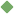factor_in 1. [ verb ] (mathematics) resolve into factors Synonyms: factor factor_out Examples: "a quantum computer can factor the number 15" Related terms: calculate arithmetic factorization To share this definition press "text" (Facebook, Twitter) or "link" (blog, mail) then paste text link Similar spelling: factor_i   factor_ii   factor_iii   factor   factoring   factor_xi   factor_ix   factor_iv   factor_xii   factor_vii   factor_xiii   factor_x   factor_viii   factor_v   factor_out   factory   factorize   factorise   factorial   faster